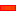﻿ Step 6D2.3: Add the Stationary Cam

# Step 6D2.3: Add the Stationary Cam

First: What do we want the Stationary Cam to look like?The image to the left shows the design we want. It has a Stationary Cam.

What is the difference between Design 1, 6D1, and this Design 2, 6D2?

In Tutorial 6D1:

 • We know the position of the sliding-part directly.

The sliding-part is a Slider. Thus, the output from, (and the input to) the Motion-Dimension FB gives the position of the Slider at its output-connector.

In Tutorial 6D2:

 • We do not know the position of the sliding-part directly.

In this case, the sliding-part is one of the Parts in an RRP Dyad. The Gear-Pair moves the Dyad.This is the Motion for the Rocker that we have prepared.

Q: How can we measure the position of the sliding-part?

A1: METHOD 1

Use a Measurement FB to measure the position [and motion] of the sliding-part relative to the Base-Part.

A2: METHOD 2

Use a Point-Data FB to give the position [and motion] of the sliding-part with mechanism coordinates.

####Method 1: Add a Measurement FB

A Measurement FB measures the distance between sketch-elements in two different Parts.

In this case, we will measure the distance from a stationary sketch-element in the Base-Part to a moving sketch-element in the sliding-part.Add a sketch-element to the Base-PartSTEP 1: Edit the Base-Part
 STEP 2: Add a vertical Line – see the image to the left.
 STEP 3: Close the Part-EditorAdd a Line to the sliding-part:

 STEP 4: Edit the sliding-part
 STEP 5: Add a vertical Line

The Line is at the Origin of the sliding-part.

 STEP 6: Close the Part-Editor

Use a Measurement FB to measure the perpendicular[⊥] distance between the Line in the Base-Part and a Point in the sliding-PartSelect the Line in the Base-Part, and then a Point at the end of the Line in the sliding-part.

 STEP 7: Add the Measurement FB between the two new sketch-elements
 STEP 8: We need to find the range of the Measurement.
 STEP 9: Add a Graph FB and drag a wire from the Measurement FB to the Graph FB.

The Measurement Range is: Minimum = 20.000mm; Maximum = 220.00mm

 STEP 10: Cycle the kinematic-chain [ALT+C]STEP 11: Add a Motion FB
 STEP 12: Double-click the Motion FB to open the dialog-box
 STEP 13: Click the box arrow and select the Rocker motion in MotionDesigner
 STEP 14: Then connect a wire from the output-connector of the Measurement FB to the Motion FB.
 STEP 15: Connect a wire from the output-connector of the Motion FB to the input-connector of the Motion-Dimension FB [Rocker].

For information: when the Measurement FB measures between two Points, the

 • TOP output gives the distance between the Points and the motion derivatives
 • BOTTOM output gives the angle between the Points and the motion derivatives.

We want the perpendicular[⊥] distance - the TOP output. See image.Click to Expand / Collapse

Look at the output from the Motion FB now we have connected the output-connector from the Measurement FB to the input-connector of the Motion FB. See the image.

The Red lines/arrows indicate the range of output from the Measurement FB (20>220>20). When we connect the Measurement FB to the Motion FB, the output from the Motion FB is limited to the motion between the Red lines/arrows along the X-axis.  That is, between 20 and 200.

Note: The UNITS of the Measurement FB Units ARE IRRELEVANT. Simply, it is the number values provided to the Motion FB that are important.STEP 16: Click the Add 2D-Cam
 STEP 17: Click the Base-Part
 STEP 18: Click the Cam-Follower Roller

MechDesigner adds the 2D-Cam to the Base-Part / Machine Frame.

We can use the same method, if we use the Point-Data FB.

Notes:

 • If the Stationary Cam has a linear motion range greater than 360 'units', edit the motion in MotionDesigner so the X-axis is greater than 360. Remember, the units of the Motion FB X-axis are dimensionless.
 • It is best to make sure the Measurement FB does not need to output a negative value.
 • You can use a Gearing FB to 'scale' or 'shift' the output of the Measurement FB to suit the motion and the requirements of the Stationary Cam.

Tutorial and Reference Help Files for MechDesigner and MotionDesigner 13.2 + © Machine, Mechanism, Motion and Cam Design Software by PSMotion Ltd